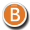• 3D Figure Assets

By:  Deva3D ()

7.50 USD
40% off• 3D Figure Assets

By:  Deva3D ()

12.50 USD• 3D Figure Assets

By:  Deva3D ()

7.50 USD
40% off• 3D Figure Assets

By:  Deva3D ()

12.50 USD• 3D Figure Assets

By:  Deva3D ()

12.50 USD• 3D Figure Assets

By:  Deva3D ()

7.50 USD
44% off• 3D Figure Assets

By:  Deva3D ()

13.50 USD• 3D Figure Assets

By:  Deva3D ()

7.77 USD
40% off• 3D Figure Assets

By:  Deva3D ()

12.95 USD• 3D Figure Assets

By:  Deva3D ()

7.00 USD
46% off• 3D Figure Assets

By:  Deva3D ()

13.50 USD• 3D Figure Assets

By:  Deva3D ()

7.00 USD
46% off• 3D Figure Assets

By:  Deva3D ()

12.50 USD• 3D Figure Assets

By:  Deva3D ()

7.50 USD
42% off• 3D Figure Assets

By:  Deva3D ()

12.50 USD• 3D Figure Assets

By:  Deva3D ()

12.95 USD• 3D Figure Assets

By:  Deva3D ()

13.00 USD• 3D Figure Assets

By:  Deva3D ()

14.49 USD• 3D Figure Assets

By:  Deva3D ()

14.49 USD• 3D Figure Assets

By:  Deva3D ()

13.50 USD• 3D Figure Assets

By:  Deva3D ()

7.95 USD
43% off• 3D Figure Assets

By:  Deva3D ()

12.99 USD• 3D Figure Assets

By:  Deva3D ()

6.45 USD
50% off• 3D Figure Assets

By:  Deva3D ()

15.99 USD• 3D Figure Assets

By:  Deva3D ()

14.95 USD• 3D Figure Assets

By:  Deva3D ()

15.75 USD• 3D Figure Assets

By:  Deva3D ()

14.85 USD• 3D Figure Assets

By:  Deva3D ()

14.99 USD• 3D Figure Assets

By:  Deva3D ()

14.99 USD• 3D Figure Assets

By:  Deva3D ()

15.55 USD• 3D Figure Assets

By:  Deva3D ()

15.39 USD• 3D Figure Assets

By:  Deva3D ()

14.49 USD• 3D Figure Assets

By:  Deva3D ()

12.49 USD• 3D Figure Assets

By:  Deva3D ()

14.49 USD• 3D Figure Assets

By:  Deva3D ()

14.95 USD• 3D Figure Assets

By:  Deva3D ()

15.95 USD• 3D Figure Assets

By:  Deva3D ()

14.49 USD• 3D Figure Assets

By:  Deva3D ()

16.95 USD• 3D Figure Assets

By:  Deva3D ()

16.95 USD• 3D Figure Assets

By:  Deva3D ()

16.95 USD• 3D Figure Assets

By:  Deva3D ()

9.72 USD
35% off